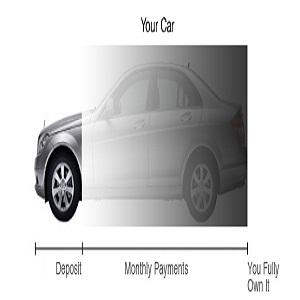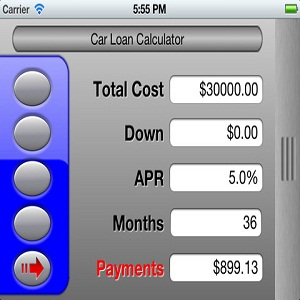# How to Calculate Vehicle Finance

Vehicle financing makes buying a vehicle possible and easier for people who cannot afford to pay the lump sum amount.  If you plan to lease a car from a bank or entered into a dealership contract with a vehicle finance company calculating the costs that come with it is the preliminary step. Knowing how to calculate car finance lets you have an idea of the money you will have to give away with time. To put crudely, vehicle ‘finance’ is the extra money that you pay for lending the amount.

### Instructions

• 1

Know the terms:

Vehicle finance usually consists of two payments: one is the down payment or deposit in the beginning and the other is the monthly payment.

The down payment is dependent on the amount of money you can afford to pay initially as a lump sum amount. This could range from ten to fifty percent of the price.

The rest of the money will be financed in monthly payments. These monthly payments are dependent on the interest charges and the duration of the contract. In the monthly payments, you not only return the monthly installments of the amount you borrowed but also the interest the bank charges you for lending the money.• 2

Principal amount:

Calculate the actual amount that you are going to borrow. If you are not making any down payment, the car price is your actual amount also known as principal amount. If you are making any down payment, this will be subtracted and the remaining amount will be the principal or financed amount. For this example, we assume a principal of 13000\$.• 3

Length of finance:

You should know the length of the money borrowed. This is typically anywhere between two to five years and even longer. You have to calculate the year length into months. In our example here, the years assumed are 5. Multiplying 5 by 12 gives us the number of months.• 4

Monthly interest rate:

Determine the monthly interest rate. This is done by dividing the annual interest rate by 12 and hundred. For an annual interest rate of 6%, the monthly rate would be 6/(12*100) giving 0.005.• 5

Calculate manually:

You can use a calculator to calculate the charges. We have used an example here to make it easier to understand.:

Banks and financing institutions use compound interest method to calculate the monthly payments.

- Add 1 to the monthly interest rate.

1+0.005 =1.005 (a)

- Now raise it to the power of months i.e 60.

(1.005) ^60 = 1.34885 (b)

- subtract 1 from (b).

1.34885 - 1 = 0.34885  (c)

- Multiply monthly interest rate (0.05) with (b) and divide the answer with (c).

(0.005 * 1.34885)/ 0.34885 = 0.019333 (d)

- Multiply (d) with the principal amount i.e. 13000 to get the monthly payment.

0.019333 * 13000= 251.329 (e)

- Multiply the monthly payment (e) with number of months to get the total amount you will pay over time.

251.329 * 60 = 15079.74

- Calculate the finance charges by subtracting the principal amount by the total payment you have to make.

15079.74 - 13000 = 2079.74• 6

Use an online calculator:

You can avoid the lengthy process above by using an online calculator available at different sites including bankrate.com and cars.com that have user friendly interface. All you have to do is to plug in your principal amount, interest rate, duration of loan and they will give you your monthly payment and total payment so you know the finance charges.

Mobile phone applications with the same attributes are available for iPhone and Android phones.• 7

Calculate finance charges using Microsoft excel:

Excel has an inbuilt finance function known as PMT. All you have to do is to type your details inside the function like this.

=PMT(interest/12*100, number of months, principal amount)

For our example it will be:

=PMT ( 6/1200, 60, -13000)

Press enter and it will give you the monthly payment amount of 251.34.•